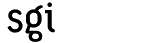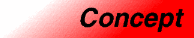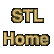# AssignableCategory: utilities Component type: concept

### Description

A type is Assignable if it is possible to copy objects of that type and to assign values to variables.

### Notation

 X A type that is a model of Assignable x, y Object of type X

### Valid expressions

Name Expression Type requirements Return type
Copy constructor X(x)   X
Copy constructor
```X x(y);
X x = y;
```

Assignment x = y    X&
Swap swap(x,y)   void

### Expression semantics

Name Expression Precondition Semantics Postcondition
Copy constructor X(x)     X(x) is a copy of x 
Copy constructor X(x)     X(x) is a copy of x 
Copy constructor
```X x(y);
X x = y;
```
x is a copy of y 
Assignment x = y      x is a copy of y 
Swap swap(x,y)   Equivalent to
```{
X tmp = x;
x = y;
y = tmp;
}
```

• int

### Notes

 One implication of this requirement is that a const type is not Assignable. For example, const int is not Assignable: if x is declared to be of type const int, then x = 7 is illegal. Similarly, the type pair<const int, int> is not Assignable.

 The reason this says "x is a copy of y", rather than "x == y", is that operator== is not necessarily defined: equality is not a requirement of Assignable. If the type X is EqualityComparable as well as Assignable, then a copy of x should compare equal to x.